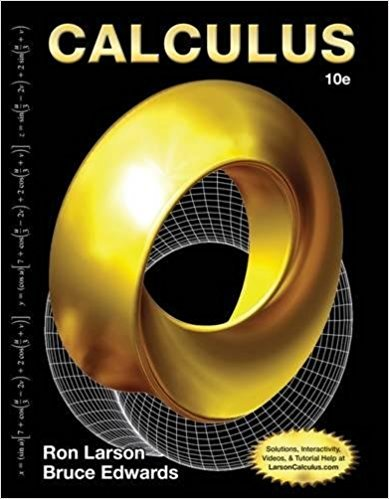×
×

# Solutions for Chapter P: Calculus 10th Edition## Full solutions for Calculus | 10th Edition

ISBN: 9781285057095Solutions for Chapter P

Solutions for Chapter P
4 5 0 263 Reviews
23
2
##### ISBN: 9781285057095

This expansive textbook survival guide covers the following chapters and their solutions. Since 15 problems in chapter P have been answered, more than 2967 students have viewed full step-by-step solutions from this chapter. Calculus was written by and is associated to the ISBN: 9781285057095. Chapter P includes 15 full step-by-step solutions. This textbook survival guide was created for the textbook: Calculus, edition: 10.

Key Calculus Terms and definitions covered in this textbook
• Blind experiment

An experiment in which subjects do not know if they have been given an active treatment or a placebo

• Coefficient matrix

A matrix whose elements are the coefficients in a system of linear equations

• Constraints

See Linear programming problem.

• Equivalent equations (inequalities)

Equations (inequalities) that have the same solutions.

• Equivalent vectors

Vectors with the same magnitude and direction.

• Inverse properties

a + 1-a2 = 0, a # 1a

• Irrational zeros

Zeros of a function that are irrational numbers.

• Linear regression line

The line for which the sum of the squares of the residuals is the smallest possible

• Mathematical induction

A process for proving that a statement is true for all natural numbers n by showing that it is true for n = 1 (the anchor) and that, if it is true for n = k, then it must be true for n = k + 1 (the inductive step)

• Multiplication property of inequality

If u < v and c > 0, then uc < vc. If u < and c < 0, then uc > vc

• n-set

A set of n objects.

• One-to-one function

A function in which each element of the range corresponds to exactly one element in the domain

• Quotient of complex numbers

a + bi c + di = ac + bd c2 + d2 + bc - ad c2 + d2 i

• Rational numbers

Numbers that can be written as a/b, where a and b are integers, and b ? 0.

• Resolving a vector

Finding the horizontal and vertical components of a vector.

• Root of a number

See Principal nth root.

• Second-degree equation in two variables

Ax 2 + Bxy + Cy2 + Dx + Ey + F = 0, where A, B, and C are not all zero.

• Sequence

See Finite sequence, Infinite sequence.

• Solve graphically

Use a graphical method, including use of a hand sketch or use of a grapher. When appropriate, the approximate solution should be confirmed algebraically

• Tree diagram

A visualization of the Multiplication Principle of Probability.

×